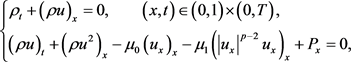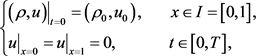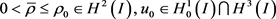﻿ 带有Power-Law型粘性项的可压缩非牛顿流的光滑解

# 带有Power-Law型粘性项的可压缩非牛顿流的光滑解Classical Solutions to the Compressible Non-Newtonian Fluids with Power-Law Viscous

Abstract: In this paper, a one-dimensional compressible non-Newtonian fluid model on a bounded interval is studied. If the initial density has a positive lower bound, the local existence of the classical solutions for the initial boundary value problem of a non-Newtonian fluid model with Power-Law viscous is proved by constructing approximate solutions and applying energy estimation.

1. 引言(1.1)(1.2)(1.3)

$\begin{array}{l}\rho \in C\left(\left[0,{T}_{*}\right];{H}^{2}\left(I\right)\right),\text{\hspace{0.17em}}{\rho }_{t}\in C\left(\left[0,{T}_{*}\right];{H}^{1}\left(I\right)\right),\text{\hspace{0.17em}}{\rho }_{tt}\in {L}^{2}\left(\left[0,{T}_{*}\right];{L}^{2}\left(I\right)\right),\rho >0,\\ {u}_{t}\in {L}^{\infty }\left(\left[0,{T}_{*}\right];{L}^{2}\left(I\right)\right),\text{\hspace{0.17em}}u\in C\left(\left[0,{T}_{*}\right];{H}_{0}^{1}\left(I\right)\cap {H}^{3}\left(I\right)\right),\\ {u}_{t}\in C\left(\left[0,{T}_{*}\right];{H}_{0}^{1}\left(I\right)\right)\cap {L}^{2}\left(\left[0,{T}_{*}\right];{H}^{2}\left(I\right)\right),\text{\hspace{0.17em}}\sqrt{\rho }{u}_{tt}\in {L}^{2}\left(\left[0,{T}_{*}\right];{L}^{2}\left(I\right)\right),\end{array}$

$\begin{array}{l}P\left(\rho \right)\in C\left(\left[0,{T}_{*}\right];{H}^{2}\left(I\right)\right),\text{\hspace{0.17em}}{P}_{t}\left(\rho \right)\in C\left(\left[0,{T}_{*}\right];{H}^{1}\left(I\right)\right),\\ {P}_{tt}\left(\rho \right)\in {L}^{2}\left(\left[0,{T}_{*}\right];{L}^{2}\left(I\right)\right),\text{\hspace{0.17em}}{\left({\mu }_{1}{|{u}_{x}|}^{p-2}{u}_{x}\right)}_{x}\in C\left(\left[0,{T}_{*}\right];{H}^{1}\left(I\right)\right).\end{array}$ (1.4)

2. 先验估计

2.1. 构造近似解

· 定义 ${u}^{0}=0$

· 对 $k\ge 1$ ，给定 ${u}^{k-1}$ ，则由  ，我们得到下列模型的光滑解 $\left({\rho }^{k},{u}^{k}\right)$

${\rho }_{t}^{k}+{u}^{k-1}{\rho }_{x}^{k}+{u}_{x}^{k-1}{\rho }^{k}=0,$ (2.1)

${\rho }^{k}{u}_{t}^{k}+{\rho }^{k}{u}^{k-1}{u}_{x}^{k}-{\mu }_{0}{u}_{xx}^{k}-{\left({\mu }_{1}{|{u}_{x}^{k}|}^{p-2}{u}_{x}^{k}\right)}_{x}+{P}_{x}\left({\rho }^{k}\right)=0,$ (2.2)

${\left({\rho }^{k},{u}^{k}\right)|}_{t=0}=\left({\rho }_{0},{u}_{0}\right),\text{\hspace{0.17em}}x\in I,\text{\hspace{0.17em}}\text{\hspace{0.17em}}\text{\hspace{0.17em}}\text{\hspace{0.17em}}{{u}^{k}|}_{x=0}={{u}^{k}|}_{x=1}=0,\text{\hspace{0.17em}}t\in \left[0,T\right].$ (2.3)

2.2. 近似解的一致估计

${M}_{0}=1+{\mu }_{0}+{\mu }_{1}+{‖{u}_{0}‖}_{{H}_{0}^{1}\left(I\right)\cap {H}^{3}\left(I\right)}+{‖{\rho }_{0}‖}_{{H}^{2}\left(I\right)}.$

$\begin{array}{l}\underset{0\le t\le {T}_{1}}{\text{esssup}}\left({‖{\rho }^{k}\left(t\right)‖}_{{H}^{1}\left(I\right)}+{‖{u}^{k}\left(t\right)‖}_{{W}_{0}^{1,p}\left(I\right)\cap {H}^{2}\left(I\right)}+{‖{u}_{t}^{k}\left(t\right)‖}_{{L}^{2}\left(I\right)}+{‖{P}_{t}\left({\rho }^{k}\right)‖}_{{L}^{2}\left(I\right)}\\ +{‖{P}_{x}\left({\rho }^{k}\right)‖}_{{L}^{2}\left(I\right)}+{‖{\rho }_{t}^{k}\left(t\right)‖}_{{L}^{2}\left(I\right)}\right)+{\int }_{0}^{{T}_{1}}{‖{u}_{xt}^{k}‖}_{{L}^{2}\left(I\right)}^{2}\text{d}s\le C\left({M}_{0}\right).\end{array}$ (2.4)

$\underset{0\le t\le {T}_{2}}{\text{esssup}}\left({‖{P}_{xx}\left({\rho }^{k}\right)‖}_{{L}^{2}\left(I\right)}+{‖{P}_{xt}\left({\rho }^{k}\right)‖}_{{L}^{2}\left(I\right)}+{‖{\rho }_{xx}^{k}‖}_{{L}^{2}\left(I\right)}+{‖{\rho }_{xt}^{k}‖}_{{L}^{2}\left(I\right)}\right)\le C\left({M}_{0}\right).$ (2.5)

${P}_{t}\left({\rho }^{k}\right)+{P}_{x}\left({\rho }^{k}\right){u}^{k-1}+\gamma P\left({\rho }^{k}\right){u}_{x}^{k-1}=0.$ (2.6)

${P}_{xt}\left({\rho }^{k}\right)+{u}^{k-1}{P}_{xx}\left({\rho }^{k}\right)+{u}_{x}^{k-1}{P}_{x}\left({\rho }^{k}\right)+\gamma {u}_{x}^{k-1}{P}_{x}\left({\rho }^{k}\right)+\gamma {u}_{xx}^{k-1}P\left({\rho }^{k}\right)=0.$ (2.7)

$\begin{array}{c}{‖{P}_{xt}\left({\rho }^{k}\right)‖}_{{L}^{2}\left(I\right)}\le {‖{u}^{k-1}‖}_{{L}^{\infty }\left(I\right)}{‖{P}_{xx}\left({\rho }^{k}\right)‖}_{{L}^{2}\left(I\right)}+{‖{u}_{x}^{k-1}‖}_{{L}^{\infty }\left(I\right)}{‖{P}_{x}\left({\rho }^{k}\right)‖}_{{L}^{2}\left(I\right)}\\ \text{\hspace{0.17em}}\text{\hspace{0.17em}}+\gamma {‖{u}_{x}^{k-1}‖}_{{L}^{\infty }\left(I\right)}{‖{P}_{x}\left({\rho }^{k}\right)‖}_{{L}^{2}\left(I\right)}+\gamma {‖{u}_{xx}^{k-1}‖}_{{L}^{2}\left(I\right)}{‖P\left({\rho }^{k}\right)‖}_{{L}^{\infty }\left(I\right)}\\ \le C\left({M}_{0}\right)\left(1+{‖{P}_{xx}\left({\rho }^{k}\right)‖}_{{L}^{2}\left(I\right)}\right).\end{array}$ (2.8)

${P}_{xxt}\left({\rho }^{k}\right)+{P}_{xxx}\left({\rho }^{k}\right){u}^{k-1}+\left(\gamma +2\right){P}_{xx}\left({\rho }^{k}\right){u}_{x}^{k-1}+\left(2\gamma +1\right){P}_{x}\left({\rho }^{k}\right){u}_{xx}^{k-1}+\gamma P\left({\rho }^{k}\right){u}_{xxx}^{k-1}=0.$ (2.9)

$\begin{array}{c}\frac{1}{2}\frac{\text{d}}{\text{d}t}{\int }_{0}^{1}{|{P}_{xx}\left({\rho }^{k}\right)|}^{2}\text{d}x\le C\left({‖{u}_{x}^{k-1}‖}_{{L}^{\infty }\left(I\right)}{‖{P}_{xx}\left({\rho }^{k}\right)‖}_{{L}^{2}\left(I\right)}^{2}\\ \text{\hspace{0.17em}}\text{\hspace{0.17em}}+{‖{P}_{x}\left({\rho }^{k}\right)‖}_{{L}^{2}\left(I\right)}{‖{P}_{xx}\left({\rho }^{k}\right)‖}_{{L}^{2}\left(I\right)}{‖{u}_{xx}^{k-1}‖}_{{L}^{\infty }\left(I\right)}\\ \text{\hspace{0.17em}}\text{\hspace{0.17em}}+{‖P\left({\rho }^{k}\right)‖}_{{L}^{\infty }\left(I\right)}{‖{P}_{xx}\left({\rho }^{k}\right)‖}_{{L}^{2}\left(I\right)}{‖{u}_{xxx}^{k-1}‖}_{{L}^{2}\left(I\right)}\right)\\ \le C{‖{P}_{xx}\left({\rho }^{k}\right)‖}_{{L}^{2}\left(I\right)}^{2}\left({‖{u}_{xxx}^{k-1}‖}_{{L}^{2}\left(I\right)}^{2}+1\right).\end{array}$ (2.10)

${u}_{xx}^{k}={\rho }^{k}{u}_{t}^{k}+{\rho }^{k}{u}^{k-1}{u}_{x}^{k}+{P}_{x}\left({\rho }^{k}\right),$ (2.11)

${\mu }_{0}|{u}_{xx}^{k}|\le |\left({\mu }_{0}+{\mu }_{1}\left(p-1\right){|{u}_{x}^{k}|}^{p-2}\right){u}_{xx}^{k}|=|{\rho }^{k}{u}_{t}^{k}+{\rho }^{k}{u}^{k-1}{u}_{x}^{k}+{P}_{x}\left({\rho }^{k}\right)|,$ (2.12)

$\begin{array}{c}{‖{I}_{1}‖}_{{L}^{2}\left(I\right)}=C{‖{|{u}_{x}^{k}|}^{p-3}{\left({u}_{xx}^{k}\right)}^{2}‖}_{{L}^{2}\left(I\right)}\\ \le C{‖{u}_{x}^{k}‖}_{{L}^{\infty }\left(I\right)}^{p-3}{‖{\left({\rho }^{k}{u}_{t}^{k}\right)}^{2}+{\left({\rho }^{k}{u}^{k-1}{u}_{x}^{k}\right)}^{2}+{\left({P}_{x}\left({\rho }^{k}\right)\right)}^{2}‖}_{{L}^{2}\left(I\right)}\\ \le C{‖{u}_{xx}^{k}‖}_{{L}^{2}\left(I\right)}^{p-3}\left({‖{\left({\rho }^{k}{u}_{t}^{k}\right)}^{2}‖}_{{L}^{2}\left(I\right)}+{‖{\left({\rho }^{k}{u}^{k-1}{u}_{x}^{k}\right)}^{2}‖}_{{L}^{2}\left(I\right)}+{‖{P}_{x}{\left(\left({\rho }^{k}\right)\right)}^{2}‖}_{{L}^{2}\left(I\right)}\right)\\ \le C\left[{‖{u}_{xt}^{k}‖}_{{L}^{2}\left(I\right)}+{‖{P}_{xx}\left({\rho }^{k}\right)‖}_{{L}^{2}\left(I\right)}+1\right],\end{array}$

$\begin{array}{c}{‖{I}_{2}‖}_{{L}^{2}\left(I\right)}=C{‖{\rho }_{x}^{k}{u}_{t}^{k}+{\rho }^{k}{u}_{xt}^{k}+{\rho }_{x}^{k}{u}^{k-1}{u}_{x}^{k}+{\rho }^{k}{u}_{x}^{k-1}{u}_{x}^{k}+{\rho }^{k}{u}^{k-1}{u}_{xx}^{k}+{P}_{xx}\left({\rho }^{k}\right)‖}_{{L}^{2}\left(I\right)}\\ \le C\left({‖{\rho }_{x}^{k}‖}_{{L}^{\infty }\left(I\right)}{‖{u}_{xt}^{k}‖}_{{L}^{2}\left(I\right)}+{‖{\rho }^{k}‖}_{{L}^{\infty }\left(I\right)}{‖{u}_{xt}^{k}‖}_{{L}^{2}\left(I\right)}+{‖{u}^{k-1}‖}_{{L}^{\infty }\left(I\right)}{‖{\rho }_{x}^{k}{u}_{x}^{k}‖}_{{L}^{2}\left(I\right)}\\ \text{\hspace{0.17em}}\text{\hspace{0.17em}}+{‖{\rho }^{k}‖}_{{L}^{\infty }\left(I\right)}{‖{u}_{x}^{k-1}{u}_{x}^{k}‖}_{{L}^{2}\left(I\right)}+{‖{\rho }^{k}‖}_{{L}^{\infty }\left(I\right)}{‖{u}^{k-1}{u}_{xx}^{k}‖}_{{L}^{2}\left(I\right)}+{‖{P}_{xx}\left({\rho }^{k}\right)‖}_{{L}^{2}\left(I\right)}\right)\\ \le C\left[{‖{u}_{xt}^{k}‖}_{{L}^{2}\left(I\right)}+{‖{P}_{xx}\left({\rho }^{k}\right)‖}_{{L}^{2}\left(I\right)}+1\right].\end{array}$

${‖{u}_{xxx}^{k}‖}_{{L}^{2}\left(I\right)}\le C\left[{‖{u}_{xt}^{k}‖}_{{L}^{2}\left(I\right)}+{‖{P}_{xx}\left({\rho }^{k}\right)‖}_{{L}^{2}\left(I\right)}+1\right].$ (2.13)

$\begin{array}{c}\underset{0\le s\le {T}_{2}}{\mathrm{sup}}{‖{P}_{xx}\left({\rho }^{k}\right)‖}_{{L}^{2}\left(I\right)}\le {‖{\rho }_{0}‖}_{{H}^{2}\left(I\right)}^{\gamma }\mathrm{exp}\left(C{\int }_{0}^{t}\left({‖{u}_{xxx}^{k-1}\left(s\right)‖}_{{L}^{2}\left(I\right)}+1\right)\text{d}s\right)\\ \le {C}_{1}\left({M}_{0}\right)\mathrm{exp}\left({C}_{2}\left({M}_{0}\right)\left({\int }_{0}^{t}{‖{P}_{xx}\left({\rho }^{k-1}\right)‖}_{{L}^{2}\left(I\right)}\text{d}s+1\right)\right).\end{array}$

$\underset{0\le s\le {T}_{2}}{\mathrm{sup}}{‖{P}_{xx}\left({\rho }^{k}\right)‖}_{{L}^{2}\left(I\right)}\le C\left({M}_{0}\right),$ (2.14)

$\underset{0\le s\le {T}_{2}}{\mathrm{sup}}{‖{P}_{xt}\left({\rho }^{k}\right)‖}_{{L}^{2}\left(I\right)}\le C\left({M}_{0}\right).$

$\underset{0\le s\le {T}_{2}}{\mathrm{sup}}\left({‖{\rho }_{xx}^{k}‖}_{{L}^{2}\left(I\right)}+{‖{\rho }_{xt}^{k}‖}_{{L}^{2}\left(I\right)}\right)\le C\left({M}_{0}\right),$ (2.15)

$\underset{0\le t\le {T}_{3}}{\text{esssup}}\left({‖{u}_{xt}^{k}‖}_{{L}^{2}\left(I\right)}^{2}+{‖{P}_{tt}\left({\rho }^{k}\right)‖}_{{L}^{2}\left(I\right)}+{‖{\rho }_{tt}^{k}‖}_{{L}^{2}\left(I\right)}\right)+{\int }_{0}^{1}\left({‖\sqrt{{\rho }^{k}}{u}_{tt}^{k}‖}_{{L}^{2}\left(I\right)}^{2}+{‖{u}_{xxt}^{k}‖}_{{L}^{2}\left(I\right)}^{2}\right)\text{d}s\le C\left({M}_{0}\right),$ (2.16)

${\Gamma }_{K}\left(t\right)=\underset{1\le k\le K}{\mathrm{max}}\underset{0\le s\le t}{\mathrm{sup}}\left(1+{‖{u}_{xt}^{k}\left(s\right)‖}_{{L}^{2}\left(I\right)}^{2}\right),$

${\rho }_{tt}^{k}+{u}^{k-1}{\rho }_{xt}^{k}+{u}_{t}^{k-1}{\rho }_{x}^{k}+{u}_{x}^{k-1}{\rho }_{t}^{k}+{u}_{xt}^{k-1}{\rho }^{k}=0,$ (2.17)

$\begin{array}{c}{‖{\rho }_{tt}^{k}‖}_{{L}^{2}\left(I\right)}^{2}\le {‖{u}^{k-1}‖}_{{L}^{\infty }\left(I\right)}^{2}{‖{\rho }_{xt}^{k}‖}_{{L}^{2}\left(I\right)}^{2}+{‖{u}_{xt}^{k-1}‖}_{{L}^{2}\left(I\right)}^{2}{‖{\rho }_{x}^{k}‖}_{{L}^{\infty }\left(I\right)}^{2}\\ \text{\hspace{0.17em}}\text{\hspace{0.17em}}+{‖{u}_{x}^{k-1}‖}_{{L}^{\infty }\left(I\right)}^{2}{‖{\rho }_{t}^{k}‖}_{{L}^{2}\left(I\right)}^{2}+{‖{u}_{xt}^{k-1}‖}_{{L}^{2}\left(I\right)}^{2}{‖{\rho }^{k}‖}_{{L}^{\infty }\left(I\right)}^{2}\\ \le C+C{‖{u}_{xt}^{k-1}‖}_{{L}^{2}\left(I\right)}^{2}+C+C{‖{u}_{xt}^{k-1}‖}_{{L}^{2}\left(I\right)}^{2}\\ \le C\left({M}_{0}\right){\Gamma }_{K}\left(t\right).\end{array}$

${‖{P}_{tt}\left({\rho }^{k}\right)‖}_{{L}^{2}\left(I\right)}^{2}\le C\left({M}_{0}\right)\left(1+{\Gamma }_{K}\left(t\right)\right).$ (2.18)

${‖{u}_{xxx}^{k}‖}_{{L}^{2}\left(I\right)}^{2}\le C\left({M}_{0}\right){\Gamma }_{K}\left(t\right).$

${\rho }_{t}^{k}{u}_{t}^{k}+{\rho }^{k}{u}_{tt}^{k}+{\rho }_{t}^{k}{u}^{k-1}{u}_{x}^{k}+{\rho }^{k}{u}_{t}^{k-1}{u}_{x}^{k}+{\rho }^{k}{u}^{k-1}{u}_{xt}^{k}-{\mu }_{0}{u}_{xxt}^{k}-{\left({\mu }_{1}{|{u}_{x}^{k}|}^{p-2}{u}_{x}^{k}\right)}_{xt}+{P}_{xt}\left({\rho }^{k}\right)=0.$ (2.19)

$\begin{array}{c}{‖{u}_{xxt}^{k}‖}_{{L}^{2}\left(I\right)}^{2}\le {‖-{\mu }_{0}{u}_{xxt}^{k}-{\mu }_{1}\left(p-1\right){\left({\left({u}_{x}^{k}\right)}^{2}\right)}^{\frac{p-2}{2}}{u}_{xxt}^{k}‖}_{{L}^{2}\left(I\right)}^{2}\\ \le {‖{\mu }_{1}\left(p-1\right)\left(p-2\right){|{u}_{x}^{k}|}^{p-2}{u}_{xt}^{k}{u}_{xx}^{k}‖}_{{L}^{2}\left(I\right)}^{2}+{‖{\rho }_{x}^{k}{u}^{k-1}{u}_{t}^{k}‖}_{{L}^{2}\left(I\right)}^{2}\\ \text{\hspace{0.17em}}\text{\hspace{0.17em}}+{‖{\rho }^{k}{u}_{x}^{k-1}{u}_{t}^{k}‖}_{{L}^{2}\left(I\right)}^{2}+{‖{\rho }^{k}{u}_{tt}^{k}‖}_{{L}^{2}\left(I\right)}^{2}+{‖{\left({\rho }^{k}{u}^{k-1}\right)}_{x}{u}^{k-1}{u}_{x}^{k}‖}_{{L}^{2}\left(I\right)}^{2}\\ \text{\hspace{0.17em}}\text{\hspace{0.17em}}+{‖{\rho }^{k}{u}_{t}^{k-1}{u}_{x}^{k}‖}_{{L}^{2}\left(I\right)}^{2}+{‖{\rho }^{k}{u}^{k-1}{u}_{xt}^{k}‖}_{{L}^{2}\left(I\right)}^{2}+{‖{P}_{xt}\left({\rho }^{k}\right)‖}_{{L}^{2}\left(I\right)}^{2}\end{array}$

$\begin{array}{l}\le C{‖{u}_{xt}^{k}‖}_{{L}^{2}\left(I\right)}^{2}{‖{u}_{xx}^{k}‖}_{{L}^{\infty }\left(I\right)}^{2}+{‖{\rho }_{x}^{k}{u}^{k-1}‖}_{{L}^{2}\left(I\right)}^{2}{‖{u}_{t}^{k}‖}_{{L}^{\infty }\left(I\right)}^{2}+{‖{\rho }^{k}{u}_{x}^{k-1}‖}_{{L}^{2}\left(I\right)}^{2}{‖{u}_{t}^{k}‖}_{{L}^{\infty }\left(I\right)}^{2}\\ \text{\hspace{0.17em}}\text{\hspace{0.17em}}+{‖\sqrt{{\rho }^{k}}‖}_{{L}^{\infty }\left(I\right)}^{2}{‖\sqrt{{\rho }^{k}}{u}_{tt}^{k}‖}_{{L}^{2}\left(I\right)}^{2}+{‖{\left({\rho }^{k}{u}^{k-1}\right)}_{x}{u}_{x}^{k}‖}_{{L}^{2}\left(I\right)}^{2}{‖{u}^{k-1}‖}_{{L}^{\infty }\left(I\right)}^{2}\\ \text{\hspace{0.17em}}\text{\hspace{0.17em}}+{‖{\rho }^{k}{u}_{x}^{k}‖}_{{L}^{2}\left(I\right)}^{2}{‖{u}_{t}^{k-1}‖}_{{L}^{\infty }\left(I\right)}^{2}+{‖{\rho }^{k}{u}^{k-1}‖}_{{L}^{\infty }\left(I\right)}^{2}{‖{u}_{xt}^{k}‖}_{{L}^{2}\left(I\right)}^{2}+{‖{P}_{xt}\left({\rho }^{k}\right)‖}_{{L}^{2}\left(I\right)}^{2}\\ \le C\left({‖{u}_{xt}^{k}‖}_{{L}^{2}\left(I\right)}^{2}+{‖{u}_{xt}^{k-1}‖}_{{L}^{2}\left(I\right)}^{2}+{‖\sqrt{{\rho }^{k}}{u}_{tt}^{k}‖}_{{L}^{2}\left(I\right)}^{2}+1\right)\\ \le C\left({M}_{0}\right)\left({\Gamma }_{K}^{2}\left(t\right)+{‖\sqrt{{\rho }^{k}}{u}_{tt}^{k}‖}_{{L}^{2}\left(I\right)}^{2}\right).\end{array}$

$\begin{array}{l}{\int }_{0}^{1}{\rho }^{k}{|{u}_{tt}^{k}|}^{2}\text{d}x+\frac{{\mu }_{0}}{2}\frac{\text{d}}{\text{d}t}{\int }_{0}^{1}{|{u}_{xt}^{k}|}^{2}\text{d}x+\frac{{\mu }_{1}\left(p-1\right)}{2}\frac{\text{d}}{\text{d}t}{\int }_{0}^{1}{|{u}_{x}^{k}|}^{p-2}{\left({u}_{xt}^{k}\right)}^{2}\text{d}x\\ =\frac{{\mu }_{1}\left(p-1\right)\left(p-2\right)}{2}{\int }_{0}^{1}{|{u}_{x}^{k}|}^{p-4}{u}_{x}^{k}{\left({u}_{xt}^{k}\right)}^{3}\text{d}x-{\int }_{0}^{1}{\rho }_{t}^{k}{u}_{t}^{k}{u}_{tt}^{k}\text{d}x-{\int }_{0}^{1}{\rho }_{t}^{k}{u}^{k-1}{u}_{x}^{k}{u}_{tt}^{k}\text{d}x\\ \text{\hspace{0.17em}}\text{\hspace{0.17em}}\text{ }-{\int }_{0}^{1}{\rho }^{k}{u}_{t}^{k-1}{u}_{x}^{k}{u}_{tt}^{k}\text{d}x-{\int }_{0}^{1}{\rho }^{k}{u}^{k-1}{u}_{xt}^{k}{u}_{tt}^{k}\text{d}x-{\int }_{0}^{1}{P}_{xt}\left({\rho }^{k}\right){u}_{tt}^{k}\text{d}x=\underset{j=1}{\overset{6}{\sum }}{I}_{j}.\end{array}$ (2.20)

$\begin{array}{c}{I}_{1}\le C{\int }_{0}^{1}{|{u}_{x}^{k}|}^{p-3}{|{u}_{xt}^{k}|}^{3}\text{d}x\le C{‖{u}_{x}^{k}‖}_{{L}^{\infty }\left(I\right)}^{p-3}{{\int }_{0}^{1}\left({u}_{xt}^{k}\right)}^{\frac{3}{2}}{\left({u}_{xt}^{k}\right)}^{\frac{3}{2}}\text{d}x\\ \le C{‖{u}_{xt}^{k}‖}_{{L}^{2}\left(I\right)}^{\frac{3}{2}}{‖{u}_{xt}^{k}‖}_{{L}^{6}\left(I\right)}^{\frac{3}{2}}\le C{‖{u}_{xt}^{k}‖}_{{L}^{2}\left(I\right)}^{\frac{3}{2}}{‖{u}_{xxt}^{k}‖}_{{L}^{2}\left(I\right)}^{\frac{3}{2}}\\ \le C{‖{u}_{xt}^{k}‖}_{{L}^{2}\left(I\right)}^{6}+\frac{1}{6}{‖{u}_{xxt}^{k}‖}_{{L}^{2}\left(I\right)}^{2}\le C\left({M}_{0}\right){\Gamma }_{K}^{3}\left(t\right)+\frac{1}{6}{‖\sqrt{{\rho }^{k}}{u}_{tt}^{k}‖}_{{L}^{2}\left(I\right)}^{2};\end{array}$

$\begin{array}{c}{I}_{2}\le -\frac{1}{2}\frac{\text{d}}{\text{d}t}{\int }_{0}^{1}{\rho }_{t}^{k}{\left({u}_{t}^{k}\right)}^{2}\text{d}x+{‖{\rho }_{tt}^{k}‖}_{{L}^{2}\left(I\right)}{‖{u}_{xt}^{k}‖}_{{L}^{2}\left(I\right)}^{2}\\ \le -\frac{1}{2}\frac{\text{d}}{\text{d}t}{\int }_{0}^{1}{\rho }_{t}^{k}{\left({u}_{t}^{k}\right)}^{2}\text{d}x+C\left({M}_{0}\right){\Gamma }_{K}^{2}\left(t\right);\end{array}$

$\begin{array}{c}{I}_{3}=-\frac{\text{d}}{\text{d}t}{\int }_{0}^{1}{\rho }_{t}^{k}{u}^{k-1}{u}_{x}^{k}{u}_{t}^{k}\text{d}x+{\int }_{0}^{1}{\rho }_{tt}^{k}{u}^{k-1}{u}_{x}^{k}{u}_{t}^{k}\text{d}x+{\int }_{0}^{1}{\rho }_{t}^{k}{u}_{t}^{k-1}{u}_{x}^{k}{u}_{t}^{k}\text{d}x+{\int }_{0}^{1}{\rho }_{t}^{k}{u}^{k-1}{u}_{xt}^{k}{u}_{t}^{k}\text{d}x\\ \le -\frac{\text{d}}{\text{d}t}{\int }_{0}^{1}{\rho }_{t}^{k}{u}^{k-1}{u}_{x}^{k}{u}_{t}^{k}\text{d}x+{‖{\rho }_{tt}^{k}‖}_{{L}^{2}\left(I\right)}{‖{u}^{k-1}‖}_{{L}^{\infty }\left(I\right)}{‖{u}^{k}‖}_{{H}^{2}\left(I\right)}{‖{u}_{xt}^{k}‖}_{{L}^{2}\left(I\right)}\\ \text{\hspace{0.17em}}\text{\hspace{0.17em}}+{‖{\rho }_{t}^{k}‖}_{{H}^{1}\left(I\right)}{‖{u}_{xt}^{k-1}‖}_{{L}^{2}\left(I\right)}{‖{u}^{k}‖}_{{H}^{2}\left(I\right)}{‖{u}_{xt}^{k}‖}_{{L}^{2}\left(I\right)}+{‖{\rho }_{t}^{k}‖}_{{L}^{2}\left(I\right)}{‖{u}^{k-1}‖}_{{L}^{\infty }\left(I\right)}{‖{u}_{xt}^{k}‖}_{{L}^{2}\left(I\right)}\\ \le -\frac{\text{d}}{\text{d}t}{\int }_{0}^{1}{\rho }_{t}^{k}{u}^{k-1}{u}_{x}^{k}{u}_{t}^{k}\text{d}x+C\left({M}_{0}\right){\Gamma }_{K}^{2}\left(t\right);\end{array}$

$\begin{array}{c}{I}_{4}\le {‖{\rho }^{k}‖}_{{L}^{\infty }\left(I\right)}^{\frac{1}{2}}{‖{u}_{xt}^{k-1}‖}_{{L}^{2}\left(I\right)}{‖{u}^{k}‖}_{{H}^{2}\left(I\right)}{‖\sqrt{{\rho }^{k}}{u}_{tt}^{k}‖}_{{L}^{2}\left(I\right)}\\ \le C\left({M}_{0}\right){\Gamma }_{k}\left(t\right)+\frac{1}{6}{‖\sqrt{{\rho }^{k}}{u}_{tt}^{k}‖}_{{L}^{2}\left(I\right)}^{2};\end{array}$

$\begin{array}{c}{I}_{5}\le {‖{\rho }^{k}‖}_{{L}^{\infty }\left(I\right)}^{\frac{1}{2}}{‖{u}^{k-1}‖}_{{L}^{\infty }\left(I\right)}{‖{u}_{xt}^{k}‖}_{{L}^{2}\left(I\right)}{‖\sqrt{{\rho }^{k}}{u}_{tt}^{k}‖}_{{L}^{2}\left(I\right)}\\ \le C\left({M}_{0}\right){\Gamma }_{k}\left(t\right)+\frac{1}{6}{‖\sqrt{{\rho }^{k}}{u}_{tt}^{k}‖}_{{L}^{2}\left(I\right)}^{2};\end{array}$

$\begin{array}{c}{I}_{6}=\frac{\text{d}}{\text{d}t}{\int }_{0}^{1}{P}_{t}\left({\rho }^{k}\right){u}_{xt}^{k}\text{d}x-{\int }_{0}^{1}{P}_{tt}\left({\rho }^{k}\right){u}_{xt}^{k}\text{d}x\\ \le \frac{\text{d}}{\text{d}t}{\int }_{0}^{1}{P}_{t}\left({\rho }^{k}\right){u}_{xt}^{k}\text{d}x+{‖{P}_{tt}\left({\rho }^{k}\right)‖}_{{L}^{2}\left(I\right)}{‖{u}_{xt}^{k}‖}_{{L}^{2}\left(I\right)}\\ \le \frac{\text{d}}{\text{d}t}{\int }_{0}^{1}{P}_{t}\left({\rho }^{k}\right){u}_{xt}^{k}\text{d}x+C\left({M}_{0}\right){\Gamma }_{K}\left(t\right).\end{array}$

$\begin{array}{l}{\int }_{0}^{1}{\rho }^{k}{\left({u}_{tt}^{k}\right)}^{2}\text{d}x+{\mu }_{0}\frac{\text{d}}{\text{d}t}{\int }_{0}^{1}{\left({u}_{xt}^{k}\right)}^{2}\text{d}x+{\mu }_{1}\left(p-1\right)\frac{\text{d}}{\text{d}t}{\int }_{0}^{1}\left({|{u}_{t}^{k}|}^{p-2}{\left({u}_{xt}^{k}\right)}^{2}\right)\text{d}x\\ \le \frac{\text{d}}{\text{d}t}{\int }_{0}^{1}\left(2{P}_{t}\left({\rho }^{k}\right){u}_{xt}^{k}-{\rho }_{t}^{k}{\left({u}_{t}^{k}\right)}^{2}-2{\rho }_{t}^{k}{u}^{k-1}{u}_{x}^{k}{u}_{t}^{k}\right)\text{d}x+C\left({M}_{0}\right){\Gamma }_{K}^{3}\left(t\right).\end{array}$ (2.21)

${‖{u}_{t}^{k}\left(0\right)‖}_{{H}_{0}^{1}\left(I\right)}\le C\left({M}_{0}\right).$

$\Lambda \left(t\right)={\int }_{0}^{1}{\mu }_{0}{|{u}_{xt}^{k}|}^{2}+{\mu }_{1}\left(p-1\right){|{u}_{x}^{k}|}^{p-2}{|{u}_{xt}^{k}|}^{2}-\left(2{P}_{t}\left({\rho }^{k}\right){u}_{xt}^{k}-{\rho }_{t}^{k}{\left({u}_{t}^{k}\right)}^{2}-2{\rho }_{t}^{k}{u}^{k-1}{u}_{x}^{k}{u}_{t}^{k}\right)\text{d}x.$

$\begin{array}{c}|\Lambda \left(t\right)|\le C\left({‖{u}_{xt}^{k}‖}_{{L}^{2}\left(I\right)}^{2}+{‖{u}_{x}^{k}‖}_{{L}^{2}\left(I\right)}^{p-2}{‖{u}_{xt}^{k}‖}_{{L}^{2}\left(I\right)}^{2}+{‖{\rho }_{t}^{k}‖}_{{L}^{2}\left(I\right)}{‖{u}_{xt}^{k}‖}_{{L}^{2}\left(I\right)}^{2}\\ \text{\hspace{0.17em}}\text{\hspace{0.17em}}+{‖{\rho }_{t}^{k}‖}_{{L}^{2}\left(I\right)}{‖{u}^{k-1}‖}_{{L}^{\infty }\left(I\right)}{‖{u}^{k}‖}_{{H}^{2}\left(I\right)}{‖{u}_{xt}^{k}‖}_{{L}^{2}\left(I\right)}+{‖{P}_{t}\left({\rho }^{k}\right)‖}_{{L}^{2}\left(I\right)}{‖{u}_{xt}^{k}‖}_{{L}^{2}\left(I\right)}\right)\\ \le C\left({‖{u}_{xt}^{k}‖}_{{L}^{2}\left(I\right)}^{2}+1\right);\end{array}$

$|\Lambda \left(t\right)|\ge \frac{1}{C}{‖{u}_{xt}^{k}‖}_{{L}^{2}\left(I\right)}^{2}-C,\text{\hspace{0.17em}}\text{\hspace{0.17em}}\text{\hspace{0.17em}}\text{\hspace{0.17em}}\text{\hspace{0.17em}}\Lambda \left(0\right)\le C\left({M}_{0}\right).$

$\underset{0\le t\le {T}_{2}}{\text{esssup}}{‖{u}_{xt}^{k}‖}_{{L}^{2}\left(I\right)}^{2}+{\int }_{0}^{t}{‖\sqrt{{\rho }^{k}}{u}_{tt}^{k}\left(t\right)‖}_{{L}^{2}\left(I\right)}^{2}\text{d}s\le C\left({M}_{0}\right)+C\left({M}_{0}\right){\int }_{0}^{t}{\Gamma }_{K}^{3}\left(t\right)\text{d}s.$ (2.22)

${\Gamma }_{K}\left(t\right)\le C\left({M}_{0}\right)\left(1+{\int }_{0}^{t}{\Gamma }_{K}^{3}\left(t\right)\right)\text{d}s,$ (2.23)

$\underset{0\le t\le {T}_{3}}{\mathrm{sup}}{\Gamma }_{K}\left(t\right)\le C\left({M}_{0}\right).$ (2.24)

$\begin{array}{l}\underset{0\le t\le {T}_{3}}{\text{esssup}}\left({‖u‖}_{{L}^{2}\left(I\right)}^{2}+{‖{P}_{tt}\left({\rho }^{k}\right)‖}_{{L}^{2}\left(I\right)}+{‖{\rho }_{tt}^{k}‖}_{{L}^{2}\left(I\right)}+{‖{u}_{xxx}^{k}‖}_{{L}^{2}\left(I\right)}\right)\\ \text{ }+{\int }_{0}^{t}\left({‖\sqrt{{\rho }^{k}}{u}_{tt}^{k}‖}_{{L}^{2}\left(I\right)}^{2}+{‖{u}_{xxt}^{k}‖}_{{L}^{2}\left(I\right)}^{2}\right)\text{d}s\le C\left({M}_{0}\right).\end{array}$ (2.25)

$\begin{array}{l}\underset{0\le t\le {T}_{*}}{\text{esssup}}\left({‖{\rho }^{k}‖}_{{H}^{1}\left(I\right)}+{‖{u}^{k}‖}_{{W}_{0}^{1,p}\left(I\right)\cap {H}^{2}\left(I\right)}+{‖{u}_{t}^{k}‖}_{{L}^{2}\left(I\right)}+{‖{P}_{t}\left({\rho }^{k}\right)‖}_{{L}^{2}\left(I\right)}+{‖{P}_{x}\left({\rho }^{k}\right)‖}_{{L}^{2}\left(I\right)}\\ \text{ }+{‖{\rho }_{t}^{k}‖}_{{L}^{2}\left(I\right)}+{‖{u}_{xt}^{k}‖}_{{L}^{2}\left(I\right)}^{2}+{‖{u}_{xxx}^{k}‖}_{{L}^{2}\left(I\right)}+{‖{P}_{xx}\left({\rho }^{k}\right)‖}_{{L}^{2}\left(I\right)}+{‖{\rho }_{xx}^{k}‖}_{{L}^{2}\left(I\right)}+{‖{P}_{xt}\left({\rho }^{k}\right)‖}_{{L}^{2}\left(I\right)}\\ \text{ }+{‖{\rho }_{xt}^{k}‖}_{{L}^{2}\left(I\right)}+{‖{P}_{tt}\left({\rho }^{k}\right)‖}_{{L}^{2}\left(I\right)}+{‖{\rho }_{tt}^{k}‖}_{{L}^{2}\left(I\right)}\right)+{\int }_{0}^{{T}_{\ast }}\left({‖\sqrt{{\rho }^{k}}{u}_{tt}^{k}‖}_{{L}^{2}\left(I\right)}^{2}+{‖{u}_{xxt}^{k}‖}_{{L}^{2}\left(I\right)}^{2}\right)\text{d}s\\ \le C\left({M}_{0}\right).\end{array}$ (2.26)

2.3. 逼近解的收敛性

${\stackrel{¯}{\rho }}^{k+1}={\rho }^{k+1}-{\rho }^{k},\text{\hspace{0.17em}}\text{\hspace{0.17em}}\text{\hspace{0.17em}}\text{\hspace{0.17em}}{\stackrel{¯}{u}}^{k+1}={u}^{k+1}-{u}^{k}.$

${\stackrel{¯}{\rho }}_{t}^{k+1}+{\left({\stackrel{¯}{\rho }}^{k+1}{u}^{k}\right)}_{x}+{\left({\rho }^{k}{\stackrel{¯}{u}}^{k}\right)}_{x}=0,$ (2.27)

$\begin{array}{l}{\rho }^{k+1}{\stackrel{¯}{u}}_{t}^{k+1}+{\rho }^{k+1}{u}^{k}{\stackrel{¯}{u}}_{x}^{k+1}+{P}_{x}\left({\rho }^{k+1}\right)-{P}_{x}\left({\rho }^{k}\right)-{\mu }_{0}{\stackrel{¯}{u}}_{xx}^{k+1}-{\mu }_{1}{\left[{|{u}_{x}^{k+1}|}^{p-2}{u}_{x}^{k+1}-{|{u}_{x}^{k}|}^{p-2}{u}_{x}^{k}\right]}_{x}\\ =-{\stackrel{¯}{\rho }}^{k+1}{u}_{t}^{k}-{\stackrel{¯}{\rho }}^{k+1}{u}^{k}{u}_{x}^{k}-{\rho }^{k}{\stackrel{¯}{u}}^{k}{u}_{x}^{k}.\end{array}$ (2.28)

${\left({\stackrel{¯}{\rho }}^{k+1},{\stackrel{¯}{u}}^{k+1}\right)|}_{t=0}=\left(0,0\right),\text{}x\in \left[0,1\right];\text{}{{\stackrel{¯}{u}}^{k+1}|}_{x=0}={{\stackrel{¯}{u}}^{k+1}|}_{x=1}=\text{0,}t\in \left[0,T\right].$ (2.29)

$\begin{array}{l}\frac{\text{d}}{\text{d}t}{‖{\stackrel{¯}{\rho }}^{k+1}‖}_{{L}^{2}\left(I\right)}^{2}\le {\int }_{0}^{t}|{\left({\stackrel{¯}{\rho }}^{k+1}\right)}_{x}{\stackrel{¯}{\rho }}^{k+1}{u}^{k}+\left({\stackrel{¯}{\rho }}^{k+1}\right){u}_{x}^{k}+{\rho }_{x}^{k}{\stackrel{¯}{u}}^{k}{\stackrel{¯}{\rho }}^{k+1}+{\rho }^{k}{\stackrel{¯}{u}}_{x}^{k}{\stackrel{¯}{\rho }}^{k+1}|\text{d}x\\ \text{}\le C\left({‖{\stackrel{¯}{\rho }}^{k+1}‖}_{{L}^{2}\left(I\right)}^{2}{‖{u}_{x}^{k}‖}_{{L}^{\infty }\left(I\right)}+{‖{\rho }^{k}‖}_{{H}^{1}\left(I\right)}{‖{u}_{x}^{k}‖}_{{L}^{2}\left(I\right)}{‖{\stackrel{¯}{\rho }}^{k+1}‖}_{{L}^{2}\left(I\right)}\right)\\ \text{}\le {C}_{\eta }{‖{\stackrel{¯}{\rho }}^{k+1}‖}_{{L}^{2}\left(I\right)}^{2}+\eta {‖{u}_{x}^{k}‖}_{{L}^{2}\left(I\right)}^{2},\end{array}$ (2.30)

$\begin{array}{l}\frac{\text{d}}{\text{d}t}{\int }_{0}^{1}{\rho }^{k+1}{|{\stackrel{¯}{u}}^{k+1}|}^{2}\text{d}x+{\int }_{0}^{1}{|{\stackrel{¯}{u}}_{x}^{k+1}|}^{2}\text{d}x\\ \le C\left({‖\sqrt{{\rho }^{k}}{\stackrel{¯}{u}}^{k}‖}_{{L}^{2}\left(I\right)}^{2}+{‖{\stackrel{¯}{\rho }}^{k+1}‖}_{{L}^{2}\left(I\right)}^{2}\right)+\frac{1}{2}{‖{u}_{x}^{k+1}‖}_{{L}^{2}\left(I\right)}^{2}.\end{array}$ (2.31)

$\begin{array}{l}\frac{\text{d}}{\text{d}t}\left({‖\sqrt{{\rho }^{k+1}}{\stackrel{¯}{u}}_{x}^{k+1}\left(t\right)‖}_{{L}^{2}\left(I\right)}^{2}+{‖{\stackrel{¯}{\rho }}^{k+1}\left(t\right)‖}_{{L}^{2}\left(I\right)}^{2}\right)+{\int }_{0}^{1}{|{\stackrel{¯}{u}}_{x}^{k+1}|}^{2}\text{d}x\\ \le C{‖\sqrt{{\rho }^{k}}{\stackrel{¯}{u}}^{k}‖}_{{L}^{2}\left(I\right)}^{2}+{C}_{\eta }{‖{\stackrel{¯}{\rho }}^{k+1}‖}_{{L}^{2}\left(I\right)}^{2}+\eta {‖{\stackrel{¯}{u}}_{x}^{k+1}‖}_{{L}^{2}\left(I\right)}^{2}.\end{array}$

$\underset{k=1}{\overset{K}{\sum }}\left[\underset{0\le t\le {T}_{*}}{\mathrm{sup}}\left({‖\sqrt{{\rho }^{k+1}}{\stackrel{¯}{u}}_{x}^{k+1}\left(t\right)‖}_{{L}^{2}\left(I\right)}^{2}+{‖{\stackrel{¯}{\rho }}^{k+1}\left(t\right)‖}_{{L}^{2}\left(I\right)}^{2}\right)+{\int }_{0}^{t}{‖{\stackrel{¯}{u}}_{x}^{k+1}\left(t\right)‖}_{{L}^{2}\left(I\right)}^{2}\text{d}s\right]\le \infty .$ (2.32)

$\begin{array}{l}\underset{0\le t\le {T}_{3}}{\text{esssup}}\left({‖\rho ‖}_{{H}^{1}\left(I\right)}+{‖u‖}_{{W}_{0}^{1,p}\left(I\right)\cap {H}^{2}\left(I\right)}+{‖{u}_{t}‖}_{{L}^{2}\left(I\right)}+{‖{P}_{t}\left(\rho \right)‖}_{{L}^{2}\left(I\right)}+{‖{P}_{x}\left(\rho \right)‖}_{{L}^{2}\left(I\right)}+{‖{\rho }_{t}‖}_{{L}^{2}\left(I\right)}\\ \text{ }+{‖{u}_{xt}‖}_{{L}^{2}\left(I\right)}^{2}+{‖{u}_{xxx}‖}_{{L}^{2}\left(I\right)}+{‖{\rho }_{xx}‖}_{{L}^{2}\left(I\right)}+{‖{P}_{xx}\left(\rho \right)‖}_{{L}^{2}\left(I\right)}+{‖{\rho }_{xt}‖}_{{L}^{2}\left(I\right)}+{‖{P}_{xt}\left(\rho \right)‖}_{{L}^{2}\left(I\right)}\\ \text{ }+{‖{\rho }_{tt}‖}_{{L}^{2}\left(I\right)}+{‖{P}_{tt}\left(\rho \right)‖}_{{L}^{2}\left(I\right)}+{\int }_{0}^{{T}_{\ast }}\left({‖\sqrt{\rho }{u}_{tt}‖}_{{L}^{2}\left(I\right)}^{2}+{‖{u}_{xxt}‖}_{{L}^{2}\left(I\right)}^{2}\right)\text{ds}\le C\left({M}_{0}\right).\end{array}$ (2.33)

3. 定理1的证明

$\rho \left(t,x\right)\ge \stackrel{¯}{\rho }\mathrm{exp}\left(-{\int }_{0}^{T}{‖{u}_{x}^{k-1}\left(x,s\right)‖}_{{L}^{\infty }\left(I\right)}\text{d}s\right)>0$ .

$\begin{array}{l}\rho \in {L}^{\infty }\left(\left[0,{T}_{\ast }\right];{H}^{2}\left(I\right)\right),{\rho }_{t}\in {L}^{\infty }\left(\left[0,{T}_{\ast }\right];{H}^{1}\left(I\right)\right),{\rho }_{tt}\in {L}^{2}\left(\left[0,{T}_{\ast }\right];{L}^{2}\left(I\right)\right),\rho >0,\\ u\in {L}^{\infty }\left(\left[0,{T}_{\ast }\right];{H}_{0}^{1}\left(I\right)\cap {H}^{3}\left(I\right)\right),{u}_{t}\in {L}^{\infty }\left(\left[0,{T}_{\ast }\right];{H}_{0}^{1}\left(I\right)\right)\cap {L}^{2}\left(\left[0,{T}_{\ast }\right];{H}^{2}\left(I\right)\right),\\ \sqrt{\rho }{u}_{tt}\in {L}^{2}\left(\left[0,{T}_{\ast }\right];{L}^{2}\left(I\right)\right),P\left(\rho \right)\in {L}^{\infty }\left(\left[0,{T}_{\ast }\right];{H}^{2}\left(I\right)\right),\\ {P}_{t}\left(\rho \right)\in {L}^{\infty }\left(\left[0,{T}_{\ast }\right];{L}^{2}\left(I\right)\right),{P}_{tt}\left(\rho \right)\in {L}^{2}\left(\left[0,{T}_{\ast }\right];{L}^{2}\left(I\right)\right).\end{array}$ (3.1)

$\rho \in C\left(\left[0,{T}_{\ast }\right];{H}^{2}\left(I\right)\right),\text{\hspace{0.17em}}\text{\hspace{0.17em}}\text{\hspace{0.17em}}\text{\hspace{0.17em}}P\left(\rho \right)\in C\left(\left[0,{T}_{\ast }\right];{H}^{2}\left(I\right)\right),$ (3.2)

${\rho }_{t}\in C\left(\left[0,{T}_{\ast }\right];{H}^{1}\left(I\right)\right),\text{\hspace{0.17em}}\text{\hspace{0.17em}}\text{\hspace{0.17em}}\text{\hspace{0.17em}}{P}_{t}\left(\rho \right)\in C\left(\left[0,{T}_{\ast }\right];{H}^{1}\left(I\right)\right).$ (3.3)

${\left(\rho u\right)}_{t}\in C\left(\left[0,{T}_{\ast }\right];{H}^{1}\left(I\right)\right).$ (3.4)

${\rho }_{tt}\in C\left(\left[0,{T}_{\ast }\right];{L}^{2}\left(I\right)\right).$ (3.5)

${\left({\mu }_{1}{|{u}_{x}|}^{p-2}{u}_{x}\right)}_{x}\in C\left(\left[0,{T}_{\ast }\right];{H}^{1}\left(I\right)\right).$ (3.6)

 Ladyzhenskaya, O.A. (1970) New Equations for the Description of the Motions of Viscous Incompressible Fluids and Global Solva-bility for Their Boundary Value Problems. Trudy Matematicheskogo Instituta imeni V. A. Steklova, 102, 85-104.

 Lions, P.L. (1998) Mathematical Topics in Fluid Mechanics: Compressible Models. Clarendon Press, Oxford.

 Necas, J. (1990) Theory of Multipolar Viscous Fluids. The Mathematics of Finite Elements and Applications, 7, 233-244.

 Necas, J. and Novotny, A. (1994) Measure-Valued Solution for Non-Newtonian Compressible Isothermal Monopolar Fluid. Acta Applicandae Mathematica, 37, 109-128.
https://doi.org/10.1007/BF00995134

 Neckcasova, S. and Lukacova, M. (1994) Bipolar Barotropic Non-Newtonian Fluid. Commentationes Mathematicae Universitatis Carolinae, 35, 467-483.

 Feireisl, E., Liao, X. and Malek, J. (2015) Global Weak Solutions to a Class of Non-Newtonian Compressible Fluids. Mathematical Methods in the Applied Sciences, 38, 3482-3494.
https://doi.org/10.1002/mma.3432

 Mamontov, A.E. (1999) Global Solvability of Multidimensional Navier-Stokes Equations of Compressible Nonlinearly Viscous Fluid. I. Siberian Mathematical Journal, 40, 351-362.
https://doi.org/10.1007/BF02679762 http://apps.webofknowledge.com/full_record.do?product=UA&search_mode=GeneralSearch&qid=4&SID=6Cb5e1i8fXVsPmEc3eL&page=1&doc=1

 Yuan, H.J. and Xu, X.J. (2008) Existence and Uniqueness of Solutions for a Class of Non-Newtonian Fluids with Singularity and Vacuum. Journal of Differential Equations, 245, 2871-2916. http://www.elsevier.com/locate/jde
https://doi.org/10.1016/j.jde.2008.04.013

 Yuan, H.J. and Li, H.P. (2012) Existence and Uniqueness of Solution for a Class of Non-Newtonian Fluids with Vacuum and Damping. Journal of Mathematical Analysis and Applications, 391, 223-239.
https://www.sciencedirect.com/science/article/pii/S0022247X12001084?via%3Dihub
https://doi.org/10.1016/j.jmaa.2012.02.015

 Fang, L. and Li, Z.L. (2015) On the Existence of Local Classical Solution for a Class of One-Dimensional Compressible Non-Newtonian Fluids. Acta Mathematica Scientia (English Series), 35, 157-181.
https://doi.org/10.1016/S0252-9602(14)60148-X

 Fang, L., Guo, Z.H. and Wang, Y.X. (2016) Local Strong Solutions to a Compressible Non-Newtonian Fluid with Density-Dependent Viscosity. Mathematical Methods in the Applied Sciences, 39, 2583-2601.
https://onlinelibrary.wiley.com/doi/pdf/10.1002/mma.3714
https://doi.org/10.1002/mma.3714

 杨迪, 佟丽宁. 真空下一维可压的非牛顿Ellis模型解的存在唯一性[J]. 应用数学与计算数学学报, 2017, 31(1): 128-142. http://qikan.cqvip.com/article/detail.aspx?id=671559827

 Yin, L., Xu, X.J. and Yuan, H.J. (2008) Global Existence and Uniqueness of Solution of the Initial Boundary Value Problem for a Class of Non-Newtianian Fluids with Vacuum. Zeitschrift fur Angewandte Mathematik und Physik, 59, 457-474.
https://doi.org/10.1007/s00033-006-5078-7

Top